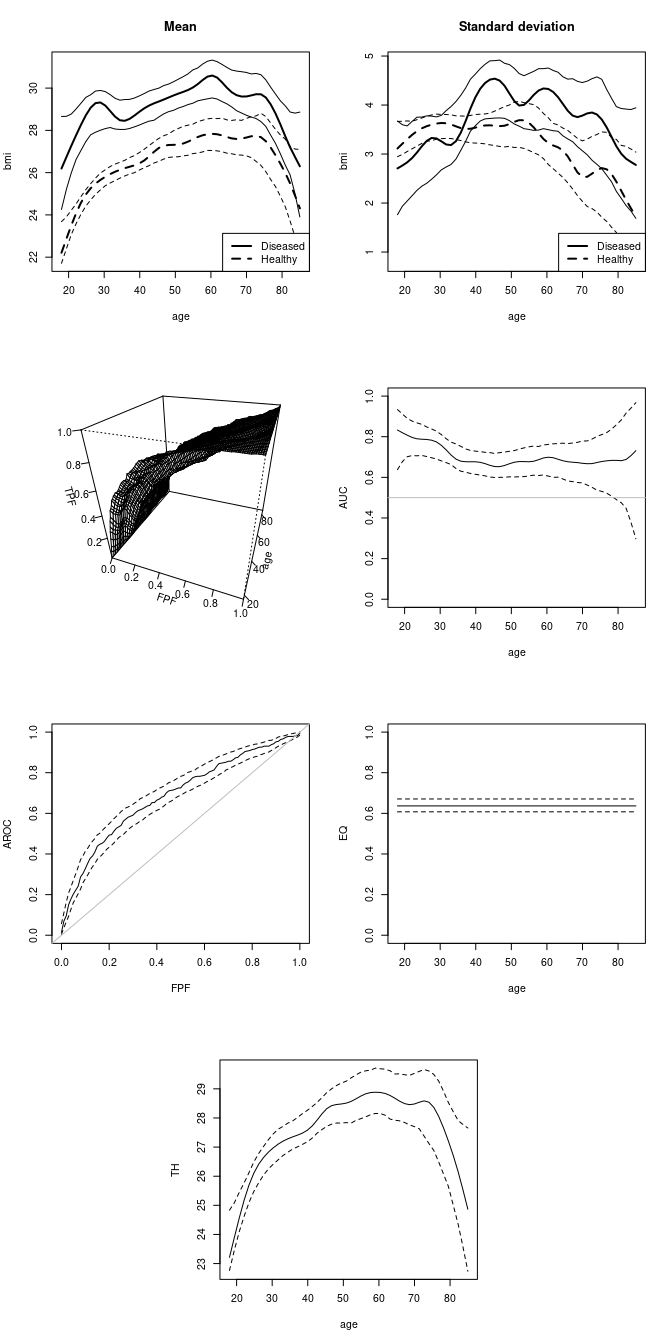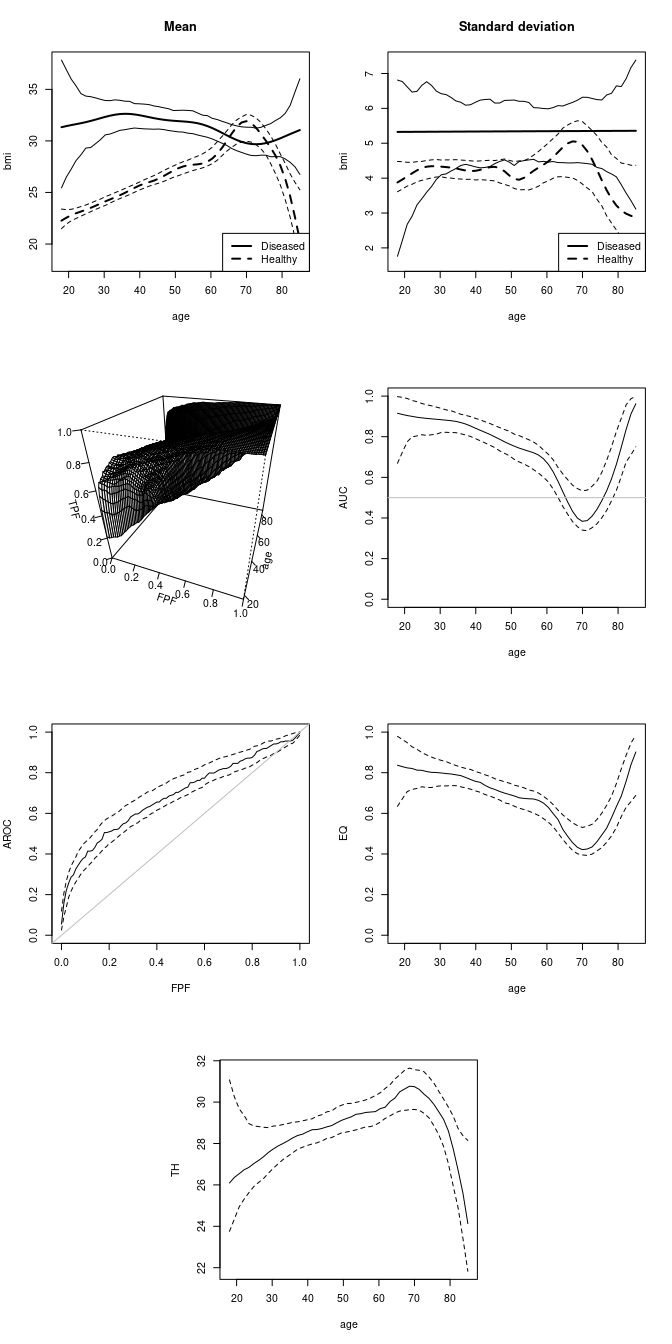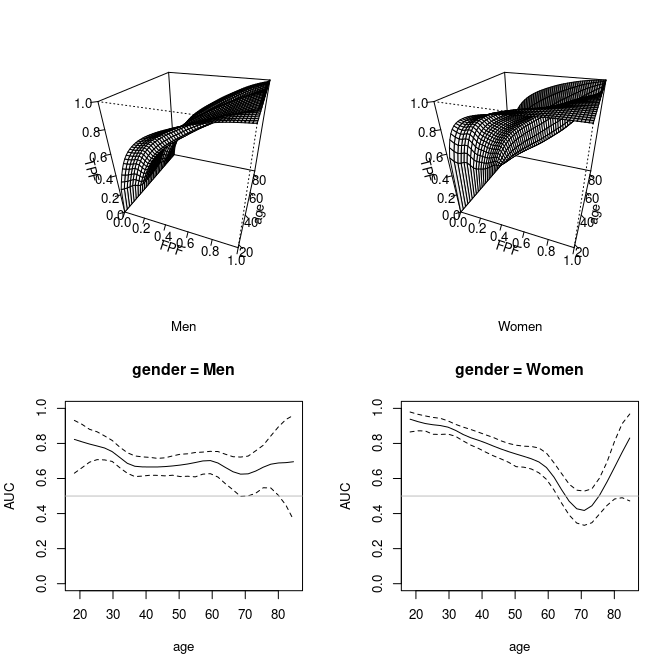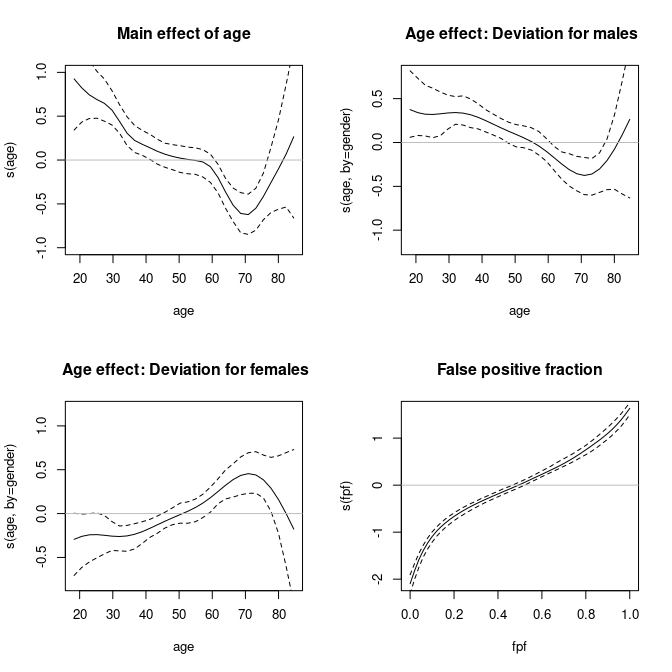# The npROCRegression package

#### 2021-11-23

This document explains the usage of the npROCRegression package. The package allows the user to apply in practice the nonparametric induced and direct ROC regression approaches presented in Rodríguez-Álvarez, Roca-Pardiñas, and Cadarso-Suárez (2011b) and Rodríguez-Álvarez, Roca-Pardiñas, and Cadarso-Suárez (2011a) respectively.

## R functions to estimate induced nonparametric ROC regression models

The main R function is INPROCreg() which estimates the covariate-specific ROC curve in the presence of a one-dimensional continuous covariate based on the induced nonparametric ROC regression approach presented in Rodríguez-Álvarez, Roca-Pardiñas, and Cadarso-Suárez (2011b), and creates an object of class INPROCreg. A brief summary of this class can be obtained by using the functions print.INPROCreg() and summary.INPROCreg(). Finally, the function plot.INPROCreg() provides automatically several plots of interest. A brief description of these functions is shown in the following Table.

Function Description
INPROCreg Fits an induced nonparametric ROC regression model for a continuous covariate.
controlINPROCreg Function used to set several parameters controlling the ROC regression fitting process.
print.INPROCreg Default print method for objects fitted with INPROCreg().
summary.INPROCreg Produces a summary of an INPROCreg object.
plot.INPROCreg Plots (a) the estimated regression and variance functions in both the healthy and diseased populations, (b) the covariate-specific ROC curve and AUC, (c) the covariate-adjusted ROC curve (AROC); and, optionally, (d) the Youden Index (YI) or the value for which the TPF and the TNF coincides (EQ); and/or (e) the optimal thresholds based on these criteria (TH)).

### INPROCreg() function

The function INPROCreg() estimates the covariate-specific ROC curve in the presence of a one-dimensional continuous covariate based on the induced nonparametric ROC regression approach presented in Rodríguez-Álvarez, Roca-Pardiñas, and Cadarso-Suárez (2011b). As a result, this function returns an object of class INPROCreg. The following Table shows a description of the arguments of this function.

Argument Description
marker A character string with the name of the diagnostic test variable.
covariate A character string with the name of the continuous covariate.
group A character string with the name of the variable that distinguishes healthy from diseased individuals.
tag.healthy The value codifying the healthy individuals in the variable group.
data Data frame representing the data and containing all needed variables.
ci.fit A logical value. If TRUE, confidence intervals are computed.
test A logical value. If TRUE, the bootstrap-based test for detecting covariate effect is performed.
accuracy A character vector indicating if the Youden index (“YI”), the value for which the TPF and the TNF coincides (“EQ”), and/or optimal threshold (“TH”) based on these two criteria should be computed.
accuracy.cal A character string indicating if the accuracy measures (argument accuracy) should be calculated based on the covariate-specific ROC curve (“ROC”) or on the covariate-adjusted ROC curve (“AROC”).
newdata A data frame containing the values of the covariate at which predictions are required
control Output of the controlINROCreg() function.
weights An optional vector of “prior weights” to be used in the fitting process.

Usage is as follows:

INPROCreg (marker, covariate, group, tag.healthy, data, ci.fit=FALSE, test=FALSE, accuracy = NULL, accuracy.cal = c("ROC","AROC"), newdata = NULL, control = controlINPROCreg(), weights=NULL)

Through marker and covariate arguments, users indicate the diagnostic test variable and the continuous covariate of interest, respectively. In group and tag.healthy arguments, we have to indicate respectively the name of the variable that distinguishes healthy from diseased individuals, and the value codifying healthy individuals in that variable. The data argument is a data frame representing the data and containing all needed variables. Bootstrap confidence intervals for the regression and variance functions, as well as for several accuracy measures, are obtained by setting the argument ci.fit to TRUE. Argument test should be set to TRUE in order to evaluate the effect of the continuous covariate on the ROC curve by means of the test presented in Rodríguez-Álvarez et al. (2016). By default, the INPROCreg() function returns the estimated regression and variance functions in both healthy and diseased populations. As far as accuracy measures is concerned, the function provides the estimated covariate-specific ROC curve, the associated covariate-specific AUCs (with the integral being approximated by numerical integration methods), and the covariate-adjusted ROC curve (AROC) (Janes and Pepe 2009). In addition, it is also possible to obtain the Youden index (“YI”), the value for which the TPF and the TNF coincides (“EQ”); and/or the optimal thresholds (“TH”) based on these two criteria (argument accuracy). Both the YI and the EQ values (and thus the optimal threshold) can be calculated based on the covariate-specific ROC curve or the AROC curve (argument accuracy.cal). It should be noted that, when a diagnostic test’s discriminatory capacity is not affected by a covariate, this does not necessarily mean that the threshold value for which optimal operational characteristics are attained will not vary with the covariate values. In such cases, the AROC curve should be used to choose the optimal TPF and TNF pairing Rodríguez-Álvarez, Roca-Pardiñas, and Cadarso-Suárez (2011b). An optional data frame containing the values of the covariate at which predictions are required can be specified in argument newdata. If this dataset is not specified, an adequate set of points from the data used in the fit is selected. A finer control of the fitting process can be achieved by the argument control, which should be the output of the function controlINPROCreg(). This function will be described later on.

We now turn to illustrate the usage of function INPROCreg() by presenting the code used in the analyses discussed in Rodríguez-Álvarez, Roca-Pardiñas, and Cadarso-Suárez (2011b) and Pardo-Fernández, Rodríguez-Álvarez, and van Keilegom (2014). For confidentiality reasons, we use here a simulated data set that resemble the original data. Specifically, in that papers we aimed at assessing the performance of the body mass index (BMI) for predicting clusters of cardiovascular disease (CVD) risk factors. Diseased subjects were defined as those having two or more CVD risk factors (raised triglycerides, reduced high-density lipoprotein cholesterol, raised blood pressure and raised fasting plasma glucose), following the International Diabetes Federation criteria (International Diabetes Federation 2006). It is well known that anthropometric measures behave differently according to both age and gender, and thus it is advisable to incorporate both covariates into the ROC analysis. Since the proposal implemented in the package only admits one continuous covariate, separate analyses were conducted on men and women.

library(npROCRegression)
data(endosim)
summary(endosim)
#>       age          gender       idf_status          bmi
#>  Min.   :18.25   Men  :1317   Min.   :0.0000   Min.   :11.53
#>  1st Qu.:29.57   Women:1523   1st Qu.:0.0000   1st Qu.:23.43
#>  Median :39.28                Median :0.0000   Median :26.59
#>  Mean   :41.43                Mean   :0.2433   Mean   :26.75
#>  3rd Qu.:50.84                3rd Qu.:0.0000   3rd Qu.:29.79
#>  Max.   :84.66                Max.   :1.0000   Max.   :51.21
#%%%%%%%%%%%%%%%%%%%%%%%%%%%%%%%%%%
# Analysis for males
#%%%%%%%%%%%%%%%%%%%%%%%%%%%%%%%%%%
fit.men <- INPROCreg(marker = "bmi", covariate = "age", group = "idf_status",
tag.healthy = 0,
data = subset(endosim, gender == "Men"),
ci.fit = TRUE, test = TRUE,
accuracy = c("EQ","TH"),
accuracy.cal="AROC",
control=controlINPROCreg(p=1,kbin=30,step.p=0.01),
newdata = data.frame(age = seq(18,85,l=50)))

#%%%%%%%%%%%%%%%%%%%%%%%%%%%%%%%%%%
# Analysis for females
#%%%%%%%%%%%%%%%%%%%%%%%%%%%%%%%%%%
fit.women <- INPROCreg(marker = "bmi", covariate = "age", group = "idf_status",
tag.healthy = 0,
data = subset(endosim, gender == "Women"),
ci.fit = TRUE, test = TRUE,
accuracy = c("EQ","TH"),
accuracy.cal="ROC",
control=controlINPROCreg(p=1,kbin=30,step.p=0.01),
newdata = data.frame(age = seq(18,85,l=50)))

As a result, the function INPROCreg() provides a list with the following components:

names(fit.men)
#>   "call"      "marker"    "covariate" "group"     "ci.fit"    "X"
#>   "fpf"       "h"         "d"         "ROC"       "AUC"       "AROC"
#>  "EQ"        "TH"        "pvalue"

where

Component Description
call The matched call.
X The data frame used in the predictions.
fpf Set of false positive fractions at which the covariate-specific ROC curve has been estimated.
h Estimated regression and variance functions in healthy population.
d Estimated regression and variance functions in diseased population.
ROC Estimated covariate-specific ROC curve.
AUC Estimated covariate-specific AUC, and corresponding confidence intervals if required.
AROC Estimated covariate-adjusted ROC curve.
YI/EQ If required, estimated covariate-specific YI (or values at which the true positive fraction (TPF) and the true negative fraction (TNF) coincide), and corresponding bootstrap confidence intervals.
TH If required, estimated optimal threshold values based on either the YI or the criterion of equality of TPF and TNF, and corresponding bootstrap confidence intervals.
pvalue If required, p-value obtained with the test for checking the effect of the continuous covariate on the ROC curve.

A numerical summary of the results can be obtained by calling up the print.INPROCreg() or INPROCreg() functions, which can be abbreviated by print() and summary():

summary(fit.men)
#>
#> Call:
#> INPROCreg(marker = "bmi", covariate = "age", group = "idf_status",
#>     tag.healthy = 0, data = subset(endosim, gender == "Men"),
#>     ci.fit = TRUE, test = TRUE, accuracy = c("EQ", "TH"), accuracy.cal = "AROC",
#>     newdata = data.frame(age = seq(18, 85, l = 50)), control = controlINPROCreg(p = 1,
#>         kbin = 30, step.p = 0.01))
#>
#> *************************************************
#> Induced non-parametric ROC regression
#> *************************************************
#> MARKER:  bmi
#> COVARIATE:  age
#> STATUS:  idf_status
#>
#> ----------------------------------------------
#> Test for continuous covariate effect (p-value): 0.0925
#>

summary(fit.women)
#>
#> Call:
#> INPROCreg(marker = "bmi", covariate = "age", group = "idf_status",
#>     tag.healthy = 0, data = subset(endosim, gender == "Women"),
#>     ci.fit = TRUE, test = TRUE, accuracy = c("EQ", "TH"), accuracy.cal = "ROC",
#>     newdata = data.frame(age = seq(18, 85, l = 50)), control = controlINPROCreg(p = 1,
#>         kbin = 30, step.p = 0.01))
#>
#> *************************************************
#> Induced non-parametric ROC regression
#> *************************************************
#> MARKER:  bmi
#> COVARIATE:  age
#> STATUS:  idf_status
#>
#> ----------------------------------------------
#> Test for continuous covariate effect (p-value): 0
#> 

### controlINPROCreg() function

The argument control of the function INPROCreg() can be used to set several parameters controlling the ROC regression fitting process. This argument is the output of the function controlINPROCreg(). This function has the following arguments:

Argument Description
step.p a numeric value, defaulting to 0.02. ROC curves are calculated at a regular sequence of false positive fractions with step.p increment.
kbin an integer value specifying the number of binning knots. By default 30.
p an integer value specifying the order of the local polynomial kernel estimator for the regression functions. By default 1.
h a vector of length 4 specifying the bandwidths to be used for the estimation of the regression and variance functions in healthy population and the regression and variance functions in diseased populations (in this order). By default -1 (selected using cross-validation.). A value of 0 would indicate a linear fit.
seed an integer value specifying the seed for the bootstrap resamples. If NULL it is initialized randomly.
nboot an integer value specifying the number of bootstrap resamples for the construction of the confidence intervals. By default 500.
level a real value specifying the confidence level for the confidence intervals. By default 0.95.
resample.m a character string specifying if bootstrap resampling (for the confidence intervals) should be done with or without regard to the disease status (“coutcome” or “noutcome”). When the resampling method is done conditionally on the disease status, the resampling is based on the residuals of the regression models in healthy and diseased populations. However, when the bootstrap resampling is done without regard to the disease status, a naive bootstrap is used. By default, the resampling is done conditionally on the disease status.

### plot.INPROCreg() function

The function plot.INPROCreg() takes as input argument an INPROCreg object, and returns several plots of interest. By default, the function returns the plot of the estimated regression and variance functions in both healthy and diseased populations, the covariate-specific ROC curve and AUC, and the AROC. When required in the call to the INPROCreg(), this plot function also returns the YI or EQ values, and corresponding optimal thresholds.

layout(matrix(c(1,1,2,2,3,3,4,4,5,5,6,6,0,7,7,0),4,4, byrow = TRUE), widths = c(1.75,1.75,1.75,1.75), heights = c(3.5,3.5,3.5,3.5))
plot(fit.men, ask = FALSE)Male population. Top row: Nonparametric estimates of BMI by age (years), along with 95% pointwise bootstrap confidence bands. Solid line: diseased population. Dashed line: healthy population. Left: Regression function. Right: Variance function. Second row: Estimated covariate-specific ROC curves and AUC along with 95% pointwise bootstrap confidence bands. Third and bottom rows: Estimated covariate-adjusted ROC curve (AROC) and estimated threshold curves for BMI (bottom panel), with the sensitivity and specificity linked to these values (right panel in third row), along with 95% pointwise bootstrap confidence bands

layout(matrix(c(1,1,2,2,3,3,4,4,5,5,6,6,0,7,7,0),4,4, byrow = TRUE), widths = c(1.75,1.75,1.75,1.75), heights = c(3.5,3.5,3.5,3.5))
plot(fit.women, ask = FALSE)Female population. Top row: Nonparametric estimates of BMI by age (years), along with 95% pointwise bootstrap confidence bands. Solid line: diseased population. Dashed line: healthy population. Left: Regression function. Right: Variance function. Second row: Estimated covariate-specific ROC curves and AUC along with 95% pointwise bootstrap confidence bands. Third and bottom rows: Estimated covariate-adjusted ROC curve (AROC) and estimated threshold curves for BMI (bottom panel), with the sensitivity and specificity linked to these values (right panel in third row), along with 95% pointwise bootstrap confidence bands

## R functions to estimate direct nonparametric ROC regression models

The main R function is DNPROCreg() which estimates the covariate-specific ROC curve in the presence of multidimensional covariates by means of the ROC-GAM regression model presented in Rodríguez-Álvarez, Roca-Pardiñas, and Cadarso-Suárez (2011a). Once the model is fitted, a brief numerical summary can be obtained by using the functions print.DNPROCreg() and summary.DNPROCreg. A plot of the estimated covariate-specific ROC curve and corresponding AUC can be obtained through the function plot.DNPROCreg(). A summary of these functions is shown in the following Table.

Function Description
DNPROCreg Fits a direct nonparametric ROC regression model for a set of continuous and categorical covariates.
controlDNPROCreg Function used to set several parameters controlling the ROC regression fitting process.
print.DNPROCreg Default print method for objects fitted with DNPROCreg().
summary.DNPROCreg Produces a summary of a DNPROCreg object.
plot.DNPROCreg Plots the covariate-specific ROC curve and AUC.
DNROCregData Selects an adequate set of points from the original data to be used as a default dataset for obtaining predictions or plots.

### DNPROCreg() function

The following Table shows a description of the arguments of the DNPROCreg() function

Argument Description
marker A character string with the name of the diagnostic test variable.
formula.h Right-hand formula(s) giving the mean and variance model(s) to be fitted in healthy population. Atomic values are also valid, being recycled.
formula.ROC Right-hand formula giving the ROC regression model to be fitted (ROC-GAM model).
group A character string with the name of the variable that distinguishes healthy from diseased individuals.
tag.healthy The value codifying the healthy individuals in the variable group.
data Data frame representing the data and containing all needed variables.
ci.fit A logical value. If TRUE, confidence intervals are computed.
test.partial A numeric vector containing the covariate components in the ROC-GAM formula to be tested for a possible effect. If NULL, no test is performed.
newdata A data frame containing the values of the covariate at which predictions are required.
control Output of the controlDNROCreg() function.
weights An optional vector of prior weights’ to be used in the fitting process.

Usage is as follows:

DNPROCreg(marker, formula.h=~1, formula.ROC=~1, group, tag.healthy, data, ci.fit=FALSE, test.partial=NULL, newdata=NULL, control=controlDNPROCreg(), weights=NULL)

The diagnostic test variable is indicated by the argument marker. The nonparametric location-scale regression model for the healthy population is specified by formula.h. This argument should be a vector (of length $$2$$) of right-hand formulas (atomic values are also valid, because they are recycled). The first right-hand formula is the model for the conditional mean function, and the second one is the model for the (logarithm) of the conditional variance function. These formulas are similar to that used for the glm() function, except that nonparametric functions can be added to the additive predictor by means of function s(). For instance, specification ~ x1 + s(x2) would assume a linear effect of x1 and a nonparametric effect of x2. Categorical variables (factors) can be also incorporated, as well as factor-by-curve interaction terms. For example, to include the interaction between age and gender we need to specify ~ gender + s(age) + s(age, by = gender). Note that, for identifiability purposes, the “main” effects of the continuous and categorical covariates need to be included into the formula. All these considerations also apply to the argument formula.ROC, where the ROC-GAM regression model is specified. The name of the variable that distinguishes healthy from diseased individuals is specified in argument group, and in tag.healthy the value codifying the healthy individuals in this variable. The data argument is a data frame representing the data and containing all needed variables. Pointwise bootstrap confidence intervals for each component of the additive predictor of the ROC-GAM, as well as the covariate-specific AUCs (with the integral being approximated by numerical integration methods), are obtained by setting the argument ci.fit to TRUE. The components of the ROC-GAM to be tested for their possible effect are indicated in test.partial. In this argument, we pass the position of the components as specified in the formula.ROC argument. An optional data frame containing the covariate values at which predictions are required can be specified in argument newdata. If missing, an adequate set of points from the dataset used in the fit is selected. To that end, the function DNPROCregdata() is used. Argument control allows to modify some default parameters that control the fitting process, and should be the output of the function controlDNPROCreg(). This function will be described later on.

To illustrate the usage of this function, we now analyse the endocrine data presented above and discussed in Rodríguez-Álvarez, Roca-Pardiñas, and Cadarso-Suárez (2011a). For the sake of illustration, in the following we will show only the statistical analysis conducted with the Body Mass Index (BMI). Since it is well established that anthropometric measures perform differently according to gender, the age-by-gender interaction was included in both the location-scale regression model for healthy population and the ROC-GAM.

library(npROCRegression)
data(endosim)

fit.endo <- DNPROCreg(marker = "bmi", formula.h = "~ gender + s(age) + s(age, by = gender)",
formula.ROC = "~ gender + s(age) + s(age, by = gender)",
group = "idf_status",
tag.healthy = 0,
data = endosim,
control = list(card.P=50, kbin=30, step.p=0.02),
ci.fit = TRUE, test.partial = 3)

As a result, the function DNPROCreg() provides a list with the following components:

names(fit.endo)
#>   "call"         "marker"       "group"        "formula.h"    "formula.ROC"
#>   "ci.fit"       "model"        "fpf"          "newdata"      "pfunctions"
#>  "coefficients" "ROC"          "AUC"          "pvalue"

where

Component Description
call The matched call.
model Data frame containing all variables and observations used in the fitting process.
fpf Set of false positive fractions at which the covariate-specific ROC curve has been estimated.
newdata Data frame containing the values of the covariates at which the covariate-specific ROC curve has been estimated.
pfunctions Matrices containing the estimates of each component of the additive predictor of the ROC-GAM. One matrix contains the effects of the covariates, the other the effect of the FPF. Confidence intervals are returned if required).
coefficients Vector of parametric coefficient of the fitted ROC-GAM.
ROC Estimated covariate-specific ROC curve.
AUC Estimated covariate-specific AUC, and corresponding confidence intervals if required.
pvalue If required, p-values are obtained - with two different bootstrap-based tests (Rodríguez-Álvarez et al. 2016) - for each model term indicated in argument test.partial (T2: $$L_{2}$$-based test; and T1: $$L_{1}$$-based test).

As before, a numerical summary of the results can be obtained by calling up the print() and summary() functions:

summary(fit.endo)
#>
#> Call:
#> DNPROCreg(marker = "bmi", formula.h = "~ gender + s(age) + s(age, by = gender)",
#>     formula.ROC = "~ gender + s(age) + s(age, by = gender)",
#>     group = "idf_status", tag.healthy = 0, data = endosim, ci.fit = TRUE,
#>     test.partial = 3, control = list(card.P = 50, kbin = 30,
#>         step.p = 0.02))
#>
#> *************************************************
#> Direct non-parametric ROC regression
#> *************************************************
#> Marker:  bmi
#> Group (status):  idf_status
#> Healthy regression model (mean):  ~gender + s(age) + s(age, by = gender)
#> Healthy regression model (variance):  ~gender + s(age) + s(age, by = gender)
#> ROC regression model:  ~gender + s(age) + s(age, by = gender)
#>
#> ----------------------------------------------
#> Parametric coefficients (ROC curve):
#>  (Intercept)   gender_Men gender_Women
#>   0.63972784  -0.06940575   0.06940575
#>
#> ----------------------------------------------
#> Tests for effects (p-values)
#>    s(age, by = gender)
#> T2                   0
#> T1                   0

### controlDNPROCreg() function

The argument control of the function DNPROCreg() can be used to set several parameters controlling the ROC regression fitting process. This argument is the output of the function controlDNPROCreg(). This function has the following arguments:

Argument Description
step.p a numeric value, defaulting to 0.02. ROC curves are calculated at a regular sequence of false positive fractions with step.p increment.
kbin an integer value specifying the number of binning knots. By default 30.
card.P an integer value specifying the cardinality of the set of false positive fractions used in the estimation process. By default 50.
p an integer value specifying the order of the local polynomial kernel estimator. By default 1.
seed an integer value specifying the seed for the bootstrap resamples. If NULL it is initialized randomly.
nboot an integer value specifying the number of bootstrap resamples for the construction of the confidence intervals. By default 500.
level a real value specifying the confidence level for the confidence intervals. By default 0.95.
resample.m a character string specifying if bootstrap resampling (for the confidence intervals) should be done with or without regard to the disease status (“coutcome” or “noutcome”). In both cases, a naive bootstrap is used. By default, the resampling is done conditionally on the disease status.
link a character string specifying the link function (“probit”, “logit” or “cloglog”). By default the link is the probit function.

### plot.DNPROCreg() function

The function plot.DNPROCreg() takes as input argument an DNPROCreg’ object, and returns the plots of the covariate-specific ROC curve and AUC.

layout(matrix(c(1,3,2,4),2,2, byrow = FALSE), widths = c(3.5,3.5), heights = c(3.5,3.5))
plot(fit.endo, ask = FALSE)Estimated covariate-specific ROC curves and AUCs, along with 95% pointwise bootstrap confidence interval, in male and female populations.

The function plot.DNPROCreg() does not plot the partial effects of the covariates on the ROC curve. However, these plots can be obtained from the element pfunctions of the DNPROCreg object.

names(fit.endo$pfunctions) #>  "covariates" "fpf" names(fit.endo$pfunctions$covariates) #>  "gender" "genderll" "genderul" #>  "s(age)" "s(age)ll" "s(age)ul" #>  "s(age, by = gender)" "s(age, by = gender)ll" "s(age, by = gender)ul" names(fit.endo$pfunctions$fpf) #>  "s(fpf)" "s(fpf)ll" "s(fpf)ul" layout(matrix(c(1,3,2,4),2,2, byrow = FALSE), widths = c(3.5,3.5), heights = c(3.5,3.5)) #%%%%%%%%%%%%%%%%%%%%%%%%%%%%%%%%%%%%%%%%%%%% # Main effect of age #%%%%%%%%%%%%%%%%%%%%%%%%%%%%%%%%%%%%%%%%%%%% sel.row <- fit.endo$newdata$gender == "Women" # Same effect for both genders plot(fit.endo$newdata$age[sel.row],fit.endo$pfunctions$covariates[sel.row, "s(age)"], xlab="age", ylab="s(age)", type="l", main = "Main effect of age", ylim=c(-1,1)) lines(fit.endo$newdata$age[sel.row], fit.endo$pfunctions$covariates[sel.row, "s(age)ul"], lty=2) lines(fit.endo$newdata$age[sel.row], fit.endo$pfunctions$covariates[sel.row, "s(age)ll"], lty=2) abline(h = 0, col="grey") #%%%%%%%%%%%%%%%%%%%%%%%%%%%%%%%%%%%%%%%%%%%% # Effect of age: deviation for males #%%%%%%%%%%%%%%%%%%%%%%%%%%%%%%%%%%%%%%%%%%%% sel.row <- fit.endo$newdata$gender == "Women" plot(fit.endo$newdata$age[sel.row], fit.endo$pfunctions$covariates[sel.row, "s(age, by = gender)"], xlab="age", ylab = "s(age, by=gender)", type = "l", main = " Age effect: Deviation for males", ylim = c(-1.2, 0.8)) lines(fit.endo$newdata$age[sel.row], fit.endo$pfunctions$covariates[sel.row, "s(age, by = gender)ul"], lty=2) lines(fit.endo$newdata$age[sel.row], fit.endo$pfunctions$covariates[sel.row, "s(age, by = gender)ll"], lty=2) abline(h = 0, col="grey") #%%%%%%%%%%%%%%%%%%%%%%%%%%%%%%%%%%%%%%%%%%%% # Effect of age: deviation for females #%%%%%%%%%%%%%%%%%%%%%%%%%%%%%%%%%%%%%%%%%%%5 sel.row <- fit.endo$newdata$gender == "Men" plot(fit.endo$newdata$age[sel.row], fit.endo$pfunctions$covariates[sel.row, "s(age, by = gender)"], xlab="age", ylab = "s(age, by=gender)", type = "l", main = " Age effect: Deviation for females", ylim = c(-0.8, 1.2)) lines(fit.endo$newdata$age[sel.row], fit.endo$pfunctions$covariates[sel.row, "s(age, by = gender)ul"], lty=2) lines(fit.endo$newdata$age[sel.row], fit.endo$pfunctions$covariates[sel.row, "s(age, by = gender)ll"], lty=2) abline(h = 0, col="grey") #%%%%%%%%%%%%%%%%%%%%%%%%%%%%%%%%%%%%%%%%%%%% # Effect of FPF #%%%%%%%%%%%%%%%%%%%%%%%%%%%%%%%%%%%%%%%%%%%5 plot(fit.endo$fpf, fit.endo$pfunctions$fpf[,1], xlab = "fpf", ylab = "s(fpf)", main = "False positive fraction", type="l")
lines(fit.endo$fpf, fit.endo$pfunctions$fpf[,2], lty=2) lines(fit.endo$fpf, fit.endo$pfunctions$fpf[,3], lty=2)
abline(h = 0, col="grey")Nonparametric estimates of partial functions (solid lines), along with 95% pointwise bootstrap confidence interval (dashed lines).

International Diabetes Federation. 2006. “The IDF Consensus Worldwide Definition of the Metabolic Syndrome.” 2006. http://www.idf.org/webdata/docs/IDF_Meta_def_final.pdf.
Janes, H., and M. S. Pepe. 2009. “Adjusting for Covariate Effects on Classification Accuracy Using the Covariate-Adjusted Receiver Operating Characteristic Curve.” Biometrika 96 (2): 371–82.
Pardo-Fernández, J., M. X. Rodríguez-Álvarez, and I. van Keilegom. 2014. “A Review on ROC Curves in the Presence of Covariates.” REVSTAT – Statistical Journal 12: 21–41.
Rodríguez-Álvarez, M. X., J. Roca-Pardiñas, and C. Cadarso-Suárez. 2011a. “A New Flexible Direct ROC Regression Model – Application to the Detection of Cardiovascular Risk Factors by Anthropometric Measures.” Computational Statistics and Data Analysis 55: 3257–70.
———. 2011b. “ROC Curve and Covariates: Extending Induced Methodology to the Non-Parametric Framework.” Statistics and Computing 21: 483–99.
Rodríguez-Álvarez, M. X., J. Roca-Pardiñas, C. Cadarso-Suárez, and P. G. Tahoces. 2016. “Boostrap-Based Procedures for Inference in Nonparametric ROC Regression Analysis.” Technical Report.